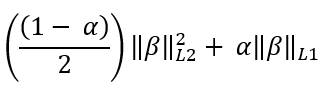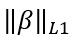# GLM2 Arguments - Teradata Vantage

## Teradata® Vantage Machine Learning Engine Analytic Function Reference

Product
Release Number
1.0
8.00
Published
May 2019
Language
English (United States)
Last Update
2019-11-22
dita:mapPath
blj1506016597986.ditamap
dita:ditavalPath
blj1506016597986.ditaval
dita:id
lmf1502735330121
Product Category
ModelTable
Specify the name for the output table that contains the trained model. The trained model contains parameters, statistics, and the coefficients of the predictors for lambda. This table must not exist.
RegularizationTable
[Optional] Specify the name for the output table that contains the statistics and coefficients of each lambda. Recommended if you want predicted results for each lambda from GLM2Predict.
InputColumns
Specify the names of the input_table columns that contain the variables to use as predictors (independent variables).
CategoricalColumns
[Optional] Specify the names of the input_table columns that contain categorical variables, and which of their categories to use in the model.
categorical_column_and_categories Description
'categorical_column:max_cardinality' Uses most common categories in categorical_column and groups other categories into category 'others'.

For example, 'column_a:3' specifies that for column_a, function uses 3 most common categories and sets category of rows that do not belong to those 3 categories to 'others'.

'categorical_column:(category [,...])' Uses specified categories of categorical_column and groups other categories into category 'others'.

For example, 'column_a : (red, yellow, blue)' specifies that for column_a, function uses categories red, yellow, and blue, and sets category of rows that do not belong to those categories to 'others'.

'categorical_column' Uses all categories in categorical_column.
If you use this argument, you must also specify each categorical_column in the InputColumns argument.
Default behavior: The function treats all variables as numerical.
For information about columns that you must identify as categorical, see Identification of Categorical Columns.
WeightColumn
[Optional] Specify the name of the input_table column that contains the weights to assign to responses.
You can use non-NULL weights to indicate that different observations have different dispersions (with the weights being inversely proportional to the dispersions). Equivalently, when the weights are positive integers wi, each response yi is the mean of wi unit-weight observations. A binomial GLM uses prior weights to give the number of trials when the response is the proportion of successes. A Poisson GLM rarely uses weights.
If the weight is less than the response value, the function throws an exception. Therefore, if the response value is greater than 1 (the default weight), you must specify a weight that is greater than or equal to the response value.
Default: 1
ResponseColumn
Specify the name of the input_table column that contains the responses.
Family
[Optional] Specify the distribution exponential family.
Default: 'GAUSSIAN'
Lambda
[Optional. Disallowed if NumLambdas is specified.] Specify the regularization parameter sequence. Each lambda must be a nonnegative DOUBLE PRECISION value. A value of zero disables regularization.
Default behavior: The function computes the regularization parameter sequence using the NumLambdas and MinLambdaRatio argument values.
NumLambdas
[Required if Lambda is omitted, disallowed otherwise] Specify the number of lambda values in the regularization parameter sequence. The num_lambdas must be a positive INTEGER. The function uses num_lambdas and min_lambda_ratio to compute the regularization parameter sequence.
Default: 100
Maximum: 10,000
MinLambdaRatio
[Required if Lambda is omitted, disallowed otherwise] Specify the minimum lambda value in the regularization parameter sequence (MinLambda) as a fraction of the maximum lambda value in the regularization parameter sequence (MaxLambda). The min_lambda_ratio must be in [0, 1).
To calculate the value of MaxLambda, the function uses the input data set.
To calculate the value of MinLambda, the function uses this formula:

MinLambda = MaxLambda * min_lambda_ratio

To calculate the step for decreasing the lambda value from MaxLambda to MinLambda, the function uses this formula:

min_lambda_ratio (1/(num_lambdas-1))

Default: 0.05 if the number of rows (observations) in the input data set is less than the number of predictors (independent variables), otherwise 0.0001.
StopThreshold
[Optional] Specify the convergence threshold of coordinate descent. The threshold must be a nonnegative DOUBLE PRECISION value.
Default: 1.0e-7
Alpha
[Optional] Specify the mixing parameter for penalty computation (see the following table). The alpha must be in [0, 1]. If alpha is in (0,1), it represents α in the elastic net regularization formula in GLM2.
alpha Regularization Type Parameter Description
0 Ridge(0,1) Elastic net1 LASSODefault: 0.1
MaxIterNum
[Optional] Specify the maximum number of iterations over the data for all lambda values. The parameter max_iterations must be a positive INTEGER value.
Default: 105
Intercept
[Optional] Specify whether the function uses an intercept. For example, in β0+β1*X1+β2*X2+ ....+ βpXp, the intercept is β0.
Default: 'true'